# Python While Loop

## Introduction

In this chapter, we will discuss while loop in Python.

## Python While Loop

A while loop is a control flow statement which checks the condition, and the condition is true then executes a block of code again and again.

Syntax:
1. while(condition):
2.         Body of the loop
Flow Chart: The flow chart of while loop is given as follows: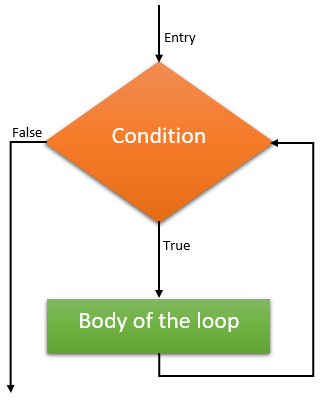In the above flow chart you can see whenever control of the program enters into the loop then, first of all on the entry time it will check some condition. If the condition will become true then only it will go inside the loop and execute the body of the loop and again it will check after first execution and if the condition is again true then it will again execute the body of the loop. This iteration goes continuously while the condition is true. Whenever the condition will be false, the control of the program comes out.

Example: Printing 1 to 20.
1. i=1
2. while(i<=20):
3.     print(i)
4.     i=i+1
Output: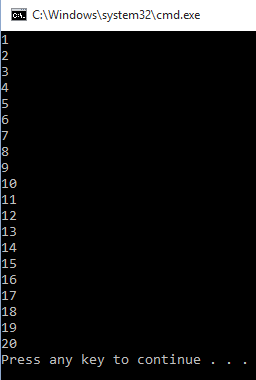Example 2: Printing table of given number.
1. num=int(input("Enter a Number : "))
2. i=1
3. while(i<=10):
4.     print(num*i)
5.     i=i+1
Output: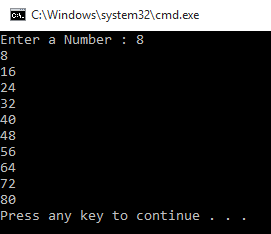Example 3:  Printing reverse of a number.
1. num = int(input("Enter a Number : "))
2. rev = 0
3. while(num != 0):
4.    rem = num % 10
5.    rev = rev * 10 + rem
6.    num = int(num / 10)
7. print(rev)
Output: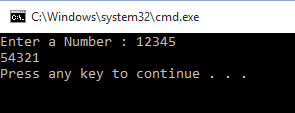### While loop with else

In python, we can also execute while loop with else part. While loop works the same as a normal while loop, but whenever while loop condition will be false it's else part will execute.

Note: else part will only execute when while loop condition will be false. If while loop will be terminated by break statement then while loop's else part never executes.

Example 4:
1. #program to check
2. #weather the given number is
3. #prime number or not
4. num = int(input("Enter a Number : "))
5. i=2;
6. while(i<num):
7.     if(num%i==0):
8.         print("%d is not a prime number..."%num)
9.         break
10.     i=i+1
11. else:
12.     print("%d is a prime number..."%num)
Output: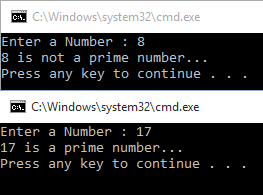## Conclusion

In the next chapter, we will learn to use Python for loop
Next » Python For Loop